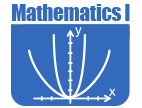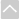# Math - Secondary Math IOER Curriculum

These Open Educational Resources are comprehensive and coherent curricular materials that may be used to teach a course or grade level.

The Math Vision Project
• Introduction to the Materials (Math 1)
Introduction to the Materials in the Mathematics One of the The MVP classroom experience begins by confronting students with an engaging task and then invites them to grapple with solving it. As students ideas emerge, take form, and are shared, the teacher orchestrates the student discussions and explorations towards a focused mathematical goal. As conjectures are made and explored, they evolve into mathematical concepts that the community of learners begins to embrace as effective strategies for analyzing and solving problems.
• Module 1: Sequences - Student Edition (Math 1)
The Mathematics Vision Project, Secondary Math One Module 1, Sequences is written as two intertwined learning cycles that begin by alternating from arithmetic sequence to geometric sequences, so students can compare and contrast features as they represent both types of sequences with tables, graphs, story contexts, diagrams, and equations.
• Module 1: Sequences - Teacher Notes (Math 1)
The Mathematics Vision Project, Secondary Math One Module 1 Teacher Notes, Sequences is written as two intertwined learning cycles that begin by alternating from arithmetic sequence to geometric sequences, so students can compare and contrast features as they represent both types of sequences with tables, graphs, story contexts, diagrams, and equations.
• Module 2: Linear & Exponential Functions - Student Edition (Math 1)
The Mathematics Vision Project, Secondary Math One Module 2, Linear and Exponential Functions, begins with a learning cycle that introduces contexts with continuous domains and defining linear functions as having a constant rate of change and exponential functions as having a constant ratio over equal intervals.
• Module 2: Linear & Exponential Functions - Teacher Notes (Math 1)
Mathematics Vision Project, Secondary Math One Module 2 Teacher Notes, Linear and Exponential Functions, begins with a learning cycle that introduces contexts with continuous domains and defining linear functions as having a constant rate of change and exponential functions as having a constant ratio over equal intervals.
• Module 3: Features of Functions - Student Edition (Math 1)
The Mathematics Vision Project, Secondary Math One Module 3, Features of Functions, is the culminating functions module in Secondary Math I. In this module, students broaden their thinking about functions to relationships that are not either linear or exponential.
• Module 3: Features of Functions - Teacher Notes (Math 1)
The Mathematics Vision Project, Secondary Math One Module 3 Teacher Notes, Features of Functions, is the culminating functions module in Secondary Math I. In this module, students broaden their thinking about functions to relationships that are not either linear or exponential.
• Module 4: Equations & Inequalities - Student Edition (Math 1)
The Mathematics Vision Project, Secondary Math One Module 4, Equations and Inequalities, students use units in combinations to define new variables for use in modeling with equations and inequalities, and interpret expressions that are the result of combining units.
• Module 4: Equations & Inequalities - Teacher Notes (Math 1)
The Mathematics Vision Project, Secondary Math One Module 4 Teacher Notes, Equations and Inequalities, students use units in combinations to define new variables for use in modeling with equations and inequalities, and interpret expressions that are the result of combining units.
• Module 5: Systems of Equations & Inequalities - Student Edition (Math 1)
The Mathematics Vision Project, Secondary Math One Module 5, Systems of Equations and Inequalities, has two learning cycles, built around a common story context that is used throughout the module. The first learning cycle begins by making the representations, tables, graphs, equations, and diagrams, needed for the rest of the module available.
• Module 5: Systems of Equations & Inequalities - Teacher Notes (Math 1)
The Mathematics Vision Project, Secondary Math One Module 5 Teacher Notes, Systems of Equations and Inequalities, has two learning cycles, built around a common story context that is used throughout the module. The first learning cycle begins by making the representations, tables, graphs, equations, and diagrams, needed for the rest of the module available.
• Module 6: Transformations & Symmetry - Student Edition (Math 1)
The Mathematics Vision Project, Secondary Math One Module 6, Transformations and Symmetry, builds on students experiences with rigid motion in earlier grades to formalize the definitions of translation, rotation, and reflection.
• Module 6: Transformations & Symmetry - Teacher Notes (Math 1)
The Mathematics Vision Project, Secondary Math One Module 6 Teacher Notes, Transformations and Symmetry, builds on students experiences with rigid motion in earlier grades to formalize the definitions of translation, rotation, and reflection.
• Module 7: Congruence, Construction & Proof - Student Edition (Math 1)
The Mathematics Vision Project, Secondary Math One Module 7, Congruence, Construction, and Proof, begins by developing constructions as another tool to be used to reason about figures and to justify properties of shapes. Individual constructions are not taught for the sake of memorizing a series of steps, but rather to reason using known properties of shapes such as circles.
• Module 7: Congruence, Construction & Proof - Teacher Notes (Math 1)
The Mathematics Vision Project, Secondary Math One Module 7 Teacher Notes, Congruence, Construction, and Proof, begins by developing constructions as another tool to be used to reason about figures and to justify properties of shapes. Individual constructions are not taught for the sake of memorizing a series of steps, but rather to reason using known properties of shapes such as circles.
• Module 8: Connecting Algebra & Geometry - Student Edition (Math 1)
The Mathematics Vision Project, Secondary Math One Module 8, Connecting Algebra and Geometry, students use the Pythagorean Theorem to find the distance between two points and to derive the distance formula.
• Module 8: Connecting Algebra & Geometry - Teacher Notes (Math 1)
The Mathematics Vision Project, Secondary Math One Module 8 Teacher Notes, Connecting Algebra and Geometry, students use the Pythagorean Theorem to find the distance between two points and to derive the distance formula.
• Module 9: Modeling Data - Student Edition (Math 1)
The Mathematics Vision Project, Secondary Math One Module 9, Modeling Data, addresses representing data in dot plots, histograms, and box plots, and analyzing the data with appropriate summary statistics for center, shape, and spread and identifying the existence of extreme data points. They compare data sets to draw conclusions and justify arguments based upon story context. This work extends the experience that students had in grades 6-8 where they informally described both center and spread.
• Module 9: Modeling Data - Teacher Notes (Math 1)
The Mathematics Vision Project, Secondary Math One Module 9 Teacher Notes, Modeling Data, addresses representing data in dot plots, histograms, and box plots, and analyzing the data with appropriate summary statistics for center, shape, and spread and identifying the existence of extreme data points. They compare data sets to draw conclusions and justify arguments based upon story context. This work extends the experience that students had in grades 6-8 where they informally described both center and spread.
• Secondary I Textbook
Secondary I Textbook is composed of modules that are aligned with the Utah Core State Standards for Mathematics. Each lesson begins with a worthwhile task that has been designed to develop mathematical understanding, solidify that understanding, or allow for practice of the new concepts, while focusing on the mathematical goals of the chosen learning cycle.
•Engage NY

• High School Mathematics
In order to assist educators with the implementation of the Common Core, the New York State Education Department provides curricular modules in Pre-K-Grade 12 English Language Arts and Mathematics that schools and districts can adopt or adapt for local purposes.Georgia Standards of Excellence Curriculum Frameworks
• Georgia Standards of Excellence Mathematics
GeorgiaStandards.Org (GSO) is a free, public website providing information and resources necessary to help meet the educational needs of students. The goal of this web site is to provide information that will enhance and support teaching and learning of Georgia standards.The Online Core Resource pages are a collaborative project between the Utah State Board of Education and the Utah Education Network. If you would like to recommend a high quality resource, contact Trish French (Elementary) or Lindsey Henderson (Secondary). If you find inaccuracies or broken links contact resources@uen.org.